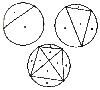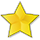BrainDen.com - Brain Teasers
• 0

# Slicing a pizza a whole different way

## Question

You want to divide a pizza into a number of slices, but you're tired of going through the center all the time. So you adopt the strategy of marking points on the circumference of the pie and making slices along all the chords that they pairwise create. At last week's pizza party you attempted to make 32 pizza chunks, from a very large pie, by this method. How many points did you have to mark off on the pizza's circumference to give you and your 15 guests at least 2 chunks each?

Bonus: The coveted bonanova gold star will be awarded if you give the formula for calculating pieces from points.

This gives you an idea:## Recommended Posts

• 0

:Make the star cut: 3 x 5 around plus the middle=16 ,

two points added cut though 5 chunks with red

cut through 7 chunks with green and cut through

4 chunks with blue.

##### Share on other sites

• 0

Firstly, you would need 6 points to split it into 32 sections.

The formula for this is 2^(# of points -1), or, more clearly, 2 raised to the power of x-1, when x is the number of points along the circumference.
##### Share on other sites

• 0

Firstly, you would need 6 points to split it into 32 sections.The formula for this is 2^(# of points -1), or, more clearly, 2 raised to the power of x-1, when x is the number of points along the circumference.

Hi cantidad and welcome to the den.

Close but that is not the answer.

##### Share on other sites

• 0

:32piz.JPG

Make the star cut: 3 x 5 around plus the middle=16 ,

two points added cut though 5 chunks with red

cut through 7 chunks with green and cut through

4 chunks with blue.

... it takes 7 points to get 32 slices.

Correct. The star awaits the formula.##### Share on other sites

• 0

:32piz.JPG

Make the star cut: 3 x 5 around plus the middle=16 ,

two points added cut though 5 chunks with red

cut through 7 chunks with green and cut through

4 chunks with blue.

... it takes 7 points to get 32 slices.

Correct. The star awaits the formula.Recursive formula

Let C(N) be maximum number of chunks as a function of the number of points N. It is easy to see that,

C(2) = 2

C(3) = 4

C(4) = 8

And a recursive expression for C(N) is

C(N) = C(N-1) + (1/6)(N-1)(N2 - 5N + 12)

Note that C(N) is the maximum number of chunks. For some N, getting the maximum number of chunks may require the points to be spaced non-equidistant from each other.

##### Share on other sites

• 0

For the max number of slices

2n - 2 n = 2 or 3

2n - 2 + SUM(I) + SUM(I) + ... + SUM(I) n > 3

Examples:

4 points gives a max of 2(4) - 2 + SUM(I) = 6 + 2 = 8

5 points gives a max of 2(5) - 2 + SUM(I) + SUM(I) = 8 + 5 + 3 = 16.

6 points gives a max of 2(6) - 2 + SUM(I) + SUM(I) + SUM(I) = 10 + 9 + 7 + 4 = 30

Maybe?

##### Share on other sites

• 0

n(n-1)(n-2)/6 + n

##### Share on other sites

• 0

I was to hasty with my previous answer.

Here's another try:

n(n-1)(n-2)(n-3)/24 + n(n-1)/2 + 1

##### Share on other sites

• 0

For the max number of slices

2n - 2 n = 2 or 3

2n - 2 + SUM(I) + SUM(I) + ... + SUM(I) n > 3

Examples:

4 points gives a max of 2(4) - 2 + SUM(I) = 6 + 2 = 8

5 points gives a max of 2(5) - 2 + SUM(I) + SUM(I) = 8 + 5 + 3 = 16.

6 points gives a max of 2(6) - 2 + SUM(I) + SUM(I) + SUM(I) = 10 + 9 + 7 + 4 = 30

Maybe?

Close, but the number that follows 16 is 31.

##### Share on other sites

• 0
I was to hasty with my previous answer.

Here's another try:

n(n-1)(n-2)(n-3)/24 + n(n-1)/2 + 1

That rings the bell. Well earned.Another form is nC4 + n-1C2 + n

•1
•1

• 0

##### Share on other sites

• 0

A problem is similar to (see my solution).

You are adding new point on the circle and drawing new chords. For each chord you count intersections with old chords and add 1, this gives you the number of new regions. This is how you arrive at the formula. Last step is to simplify it.

But to be honest I just guessed the solutionI took the formula from above mentioned problem and made an adjustment for that extra 1s. Few tries and it worked.

Edit: Guessing helped me only in the last step (simplification). I needed non-simplified formula to verify guessed one.

Edited by witzar

## Join the conversation

You can post now and register later. If you have an account, sign in now to post with your account.×   Pasted as rich text.   Paste as plain text instead

Only 75 emoji are allowed.

×   Your previous content has been restored.   Clear editor

×   You cannot paste images directly. Upload or insert images from URL.